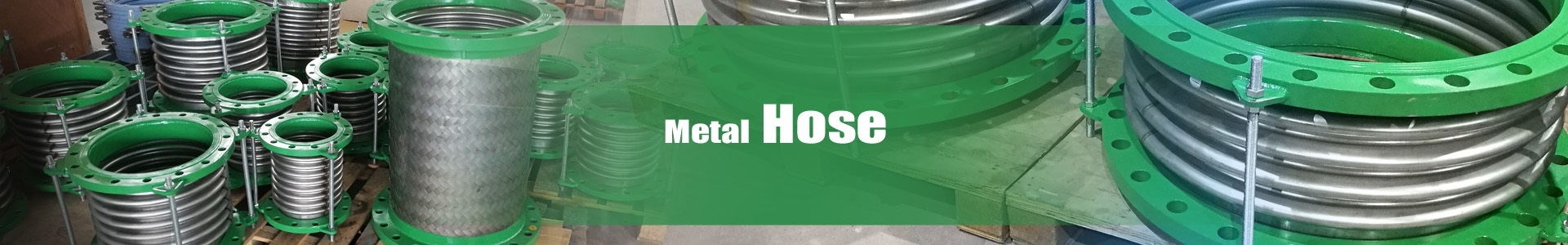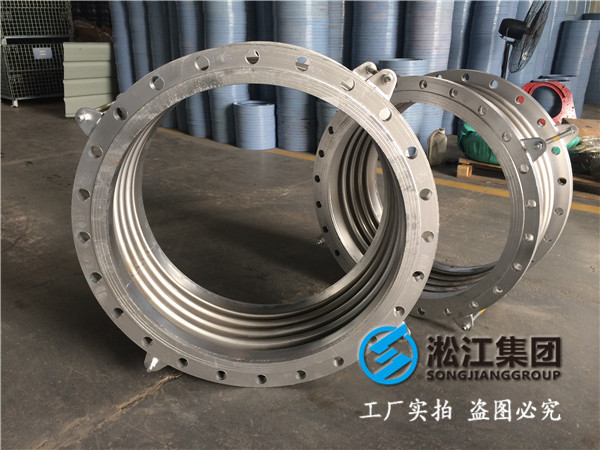# 波纹金属软管补偿器的应力与补偿量计算

编辑：淞江集团董帝豪（图片为随机配图，以专业人士为准）

管道的热变形计算
计算公式：X=a·L·△T
x 管道膨胀量
a为线膨胀系数，取0.0133mm/m
L补偿管线(所需补偿管道固定支座间的距离)长度
△T为温差(介质温度-安装时环境温度)
波纹管补偿器作用管道支座受力的计算：
内压推力：F=100·P·A=100×0.6×2445=14600(N)
轴向弹力：Fx=Kx·(f·X)=282×(1/2×32)=4512(N)
横向弹力：Fy=Ky·Y=1528×2.8=4278.4(N)
弯 矩：My=Fy·L=4278.4×4=17113.6(N·m)
Mθ=Kθ·θ =197×1.8=354.6(N·m)
合成弯矩：M=My+Mθ=17113.6+354.6=17468.2(N·m)
以上是波纹管补偿器的受力计算。